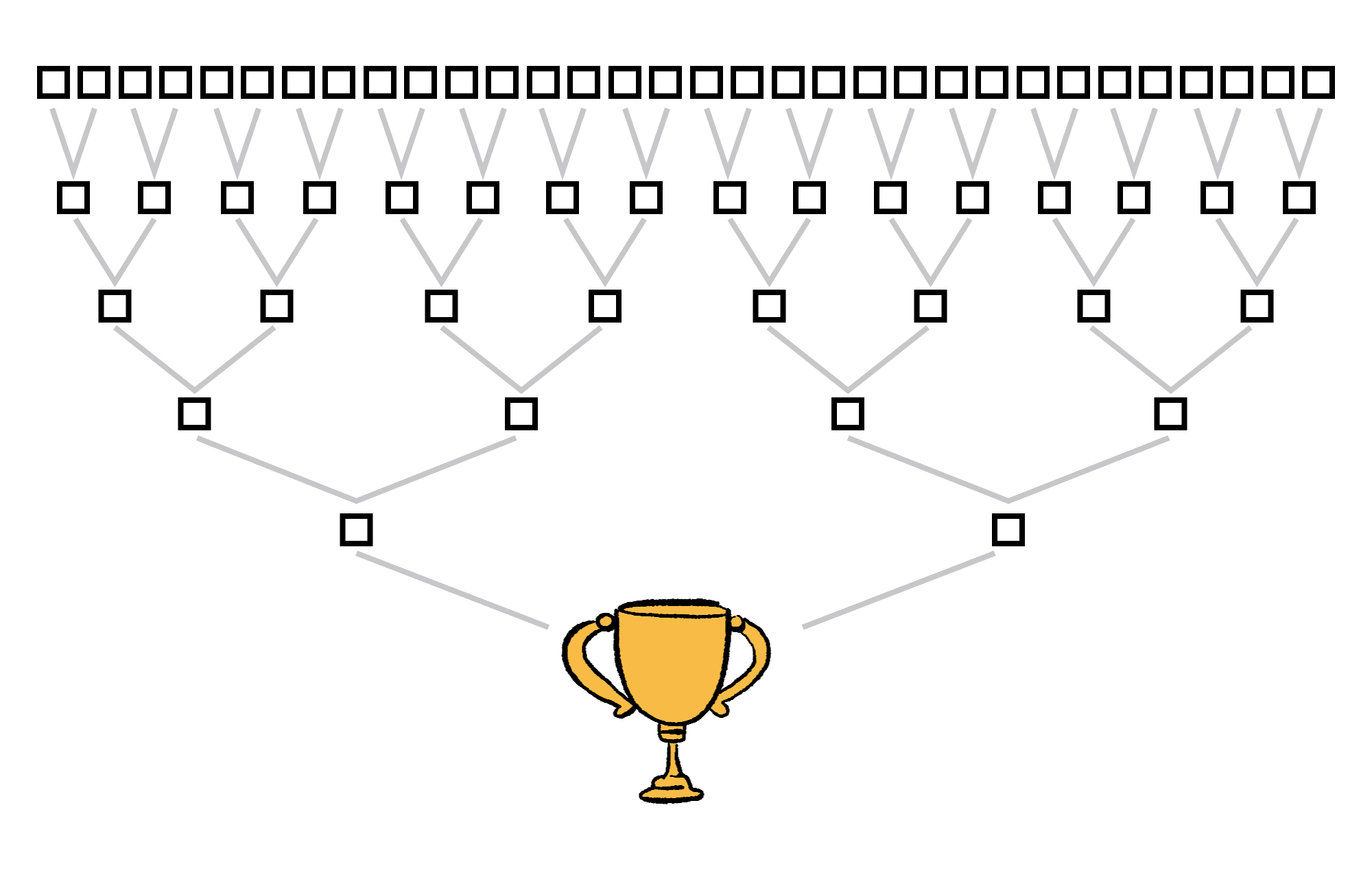## Is There a Simpler Way?

Some problems become much simpler if we look at them a different way. Let's look at a single-elimination tournament with $32$ teams. If we want to find out how many games there will be in the tournament, we could begin by thinking through the whole thing.

All the teams play, the winners advance, and this repeats until only one team is left.Since there are $32$ teams, we must have $16$ games in the first round, $8$ in the second, $4$ in the third, then $2,$ and then $1$ final game. Adding all of these up, we get $16+8+4+2+1=31$. So, it takes $31$ games to finish a single-elimination tournament with $32$ teams.

But wait… we could have done this differently. Our reasoning could have been as simple as this:

There must be $31$ games so that every team except the tournament winner can lose exactly once.

No further calculation needed.

Here are the relevant facts used in this analysis:

• Each game produces exactly one winner and one loser.
• Every team — except the tournament winner — has to lose exactly once.
• $31$ teams get eliminated.

Therefore, there must be $31$ games so that every team but the tournament winner can lose exactly once.

The problem below is extremely tedious to calculate step by step. However, if you can come up with a way to think about it differently, it will unravel in much the same way as the tournament problem above.

# Today's Challenge

Compute

$\large 2^{1000}-2^{999}-2^{998} - \cdots -2^{0} .$